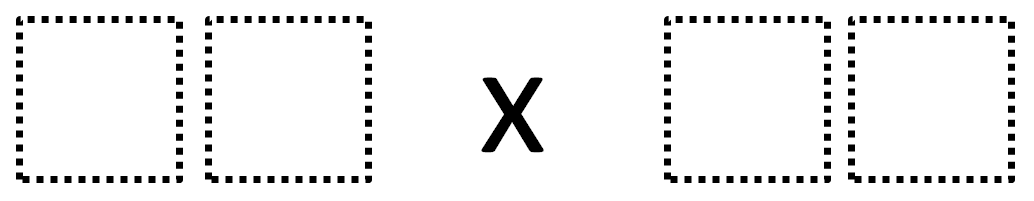Home > Grade 4 > Multiplying Two-Digit Numbers (Elementary)

# Multiplying Two-Digit Numbers (Elementary)

Directions: Make the smallest (or largest) product by filling in the boxes using the whole numbers 1-9 no more than one time each### Hint

What does the number on the left represent?  What does the number on the right represent?

96 · 87 is one answer for the largest product.  13 · 24 is the smallest product.

Source: Robert Kaplinsky

## Multiplying Differences

Directions: Using the digits 1 to 9, at most one time each, fill in the …

1.•That’s pretty close Alex! Can you make it any larger?

2.Here is a Desmos visual that might support the task.

https://www.desmos.com/calculator/44rd3rwkpk

•Robert Kaplinsky

Nice Rusty! Too bad there isn’t a built in way to restrict duplicate numbers. Two thoughts:
– I wonder if there is a difference between a horizontal and vertical representation?
– Is there value in restricting a and b to 12 to 98? (11 or less and 99 and 100 are not possible)

3.Raiden From 3rd Grade

I Did 12×5 I Dint Try For Largest Or Smallest My Whole Answer Was This:
12+12+12+12+12= 60
24 24 12=60
24+24+12=60
48+12=60